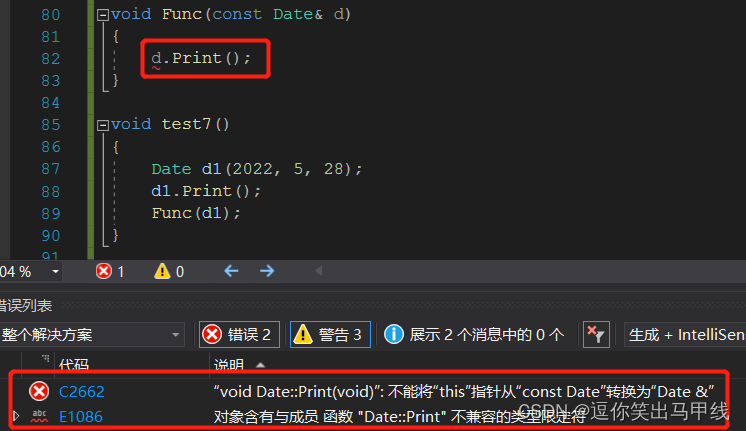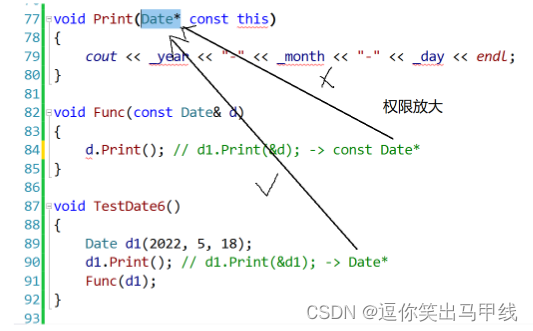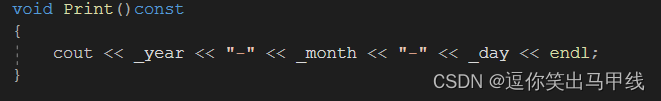> 文档中心 > 机械转码日记【14】C++运算符重载的应用——实现一个日期类计算器

# 机械转码日记【14】C++运算符重载的应用——实现一个日期类计算器

1.运算符重载

2.赋值重载函数：operator=

2.1不写赋值重载函数，编译器会默认生成

3.实现日期计算器

3.1日期类的构造函数

3.2函数复用定义">",">=","<","<=","==","!="

3.2.1复用"<","=="去实现"<="

3.2.2复用""

3.2.3复用"="

3.2.4复用"==",去实现"!="

3.3"+"和"+="

3.3.1"+"

3.3.2分清楚拷贝构造函数和赋值重载函数

3.3.3为什么赋值重载和拷贝构造函数里面的参数都建议用const修饰

3.3.4"+="

3.3.5+和+=互相复用的优劣

3.4"-"和"-="

3.5前置++，--和后置++，--

3.6"-"的另外一种重载形式，日期对象-日期对象

3.7const修饰成员

# 1.运算符重载

``bool operator<(const Date& d){if (_year < d._year|| (_year == d._year && _month < d._month)|| (_year == d._year && _month == d._month && _day < d._day)){return true;}else{return false;}}``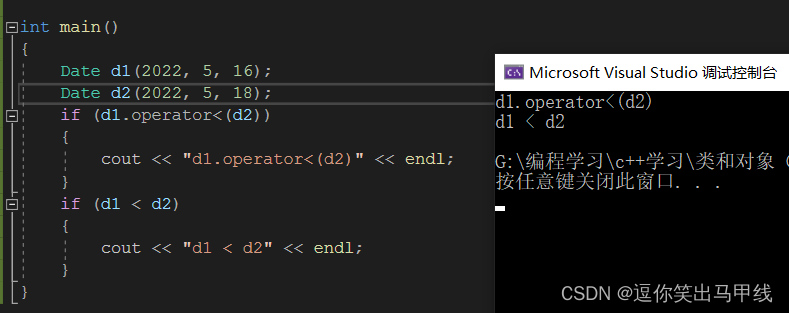• 以我们调用对象的成员函数的形式调用：d1.operator<(d2)
• 直接写成d1<d2，在这里编译器会自动给我们处理

.*::sizeof?:.    注意以上5个运算符不能重载！

# 2.赋值重载函数：operator=

``class Date{public://普通构造Date(int year = 1, int month = 1, int day = 1){_year = year;_month = month;_day = day;}    //赋值重载    void operator=(const Date& d){_year = d._year;_month = d._month;_day - d._day;}private:int _year; // 年int _month; // 月int _day; // 日};``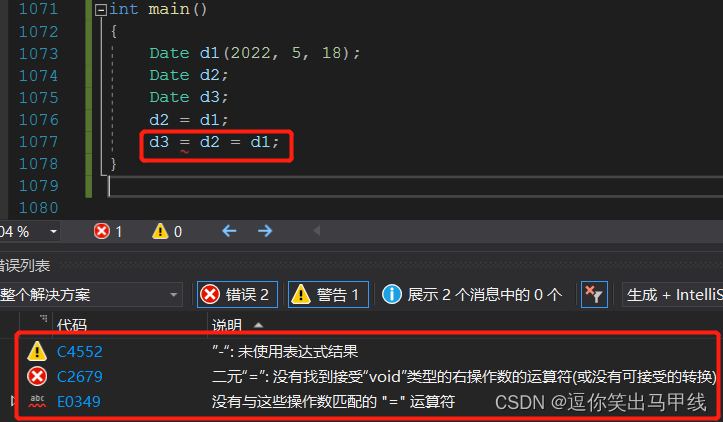`` //赋值重载    Date operator=(const Date& d){_year = d._year;_month = d._month;_day - d._day; return *this;}``

`` //赋值重载    Date& operator=(const Date& d){_year = d._year;_month = d._month;_day - d._day; return *this;}``

``//赋值重载Date& operator=(const Date& d){if (this != &d){_year = d._year;_month = d._month;_day - d._day;}return *this;}``

## 2.1不写赋值重载函数，编译器会默认生成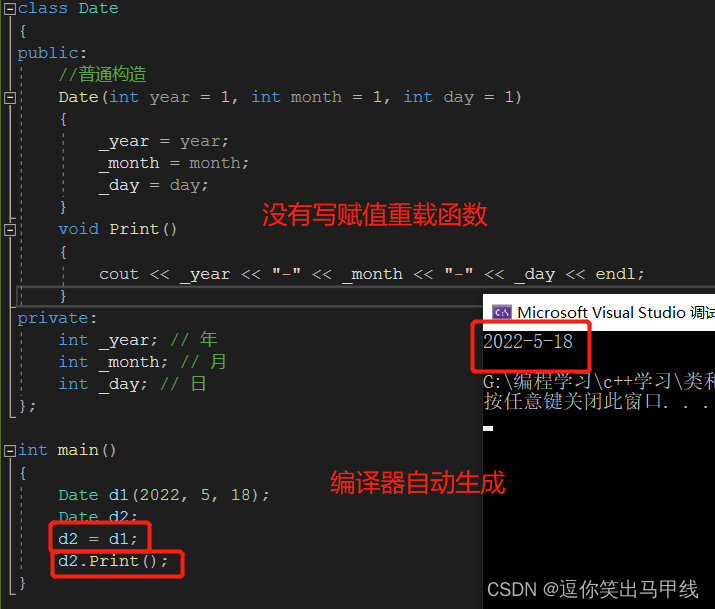# 3.实现日期计算器``#pragma once#include#include//项目里面尽量不要全展开，防止命名冲突using std::cout;using std::cin;using std::endl;class Date{public://四年一闰，百年不闰，四百年一闰bool isLeapYear(int year){return (year % 4 == 0 && year % 100 != 0) || (year % 400 == 0);}int GetMonthDay(int year, int month);Date(int year = 1, int month = 1, int day = 1);//拷贝构造和赋值不需要写，因为浅拷贝足够了//Date(const Date& d);//Date& operator=(const Date& d);void Print(){cout << _year << "-" << _month << "-" << _day << endl;}Date operator+(int day);Date& operator+=(int day);Date operator-(int day);Date& operator-=(int day);// ++d1Date& operator++();// 前置// d1++Date operator++(int);// 后置Date& operator--();// 前置Date operator--(int);// 后置// d1 - d2int operator-(const Date& d);bool operator==(const Date& d);bool operator(const Date& d);bool operator>=(const Date& d);bool operator!=(const Date& d);// d1 <= d2bool operator<=(const Date& d);private:int _year;int _month;int _day;};``

## 3.1日期类的构造函数

``//判断闰年的函数：四年一闰，百年不闰，四百年一闰bool isLeapYear(int year){return (year % 4 == 0 && year % 100 != 0) || (year % 400 == 0);}//获取当前月的天数的函数：int Date::GetMonthDay(int year, int month){assert(year >= 0 && month > 0 && month < 13);//static，因为它频繁调用，所以加上static就可以节约内存//多线程读取数据是没问题的const static int monthDayArray = { 0, 31, 28, 31, 30, 31, 30, 31, 31, 30, 31, 30, 31 };if (month == 2 && isLeapYear(year)){return 29;}else{return monthDayArray[month];}}``

``//构造函数，声明定义分离//声明给了缺省，定义就不用给了Date::Date(int year, int month, int day){if (year>0 && month <= 12 && day  0 && day > 0){_year = year;_month = month;_day = day;}else{cout << "构造失败" << endl;}}``

## 3.2函数复用定义">",">=","<","<=","==","!="

``//能复用的情况尽可能复用bool Date:: operator<(const Date& d)  {if( (_year == d._year && _month == d._month && _day < d._day)|| (_year == d._year && _month < d._month)|| (_year < d._year)){return true;}else{return false;}}bool Date:: operator==(const Date& d)  {return _year == d._year&& _month == d._month&& _day == d._day;}``

### 3.2.1复用"<","=="去实现"<="

``//复用"<","=="去实现"<="bool operator<=(const Date& d) {return *this < d || *this == d;}``

### 3.2.2复用""

``//复用""bool operator>(const Date& d){return !(*this <= d);}``

### 3.2.3复用"="

``//复用"="bool operator>=(const Date& d) {return !(*this < d);}``

### 3.2.4复用"==",去实现"!="

``//复用"==",去实现"！="bool operator!=(const Date& d)  {return !(*this == d);}``

## 3.3"+"和"+="

### 3.3.1"+"

``Date Date::operator+(int day){Date ret(*this);//需要返回临时变量，防止原来的值被修改ret._day += day;while (ret._day > GetMonthDay(ret._year, ret._month)){ret._day -= GetMonthDay(ret._year, ret._month);ret._month++;if (ret._month == 13){++ret._year;ret._month = 1;}}return ret;}``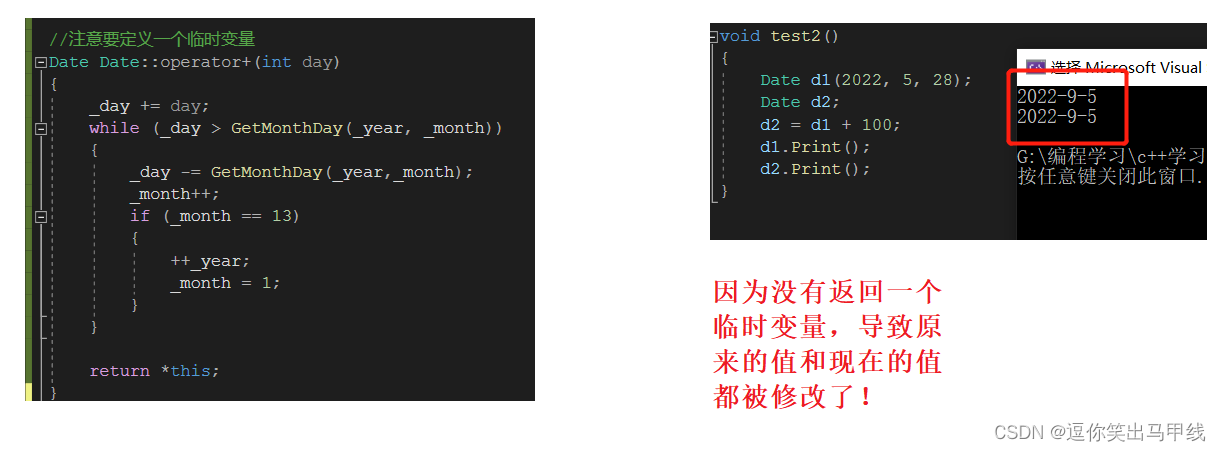### 3.3.2分清楚拷贝构造函数和赋值重载函数

``void test2(){Date d1(2022, 5, 28);cout << endl;Date d2 = d1+100;//这里是拷贝还是赋值呢？cout << endl;Date d3;cout << endl;d3 = d1;//这里是拷贝还是赋值呢？}``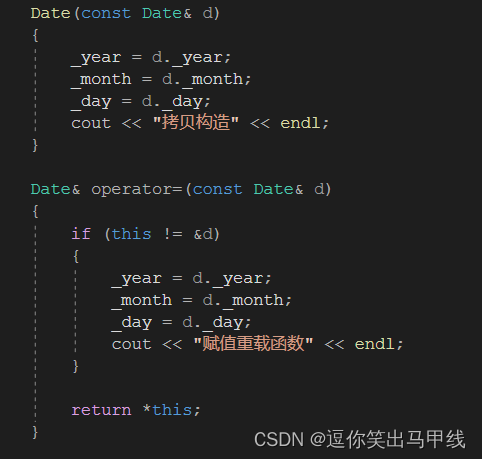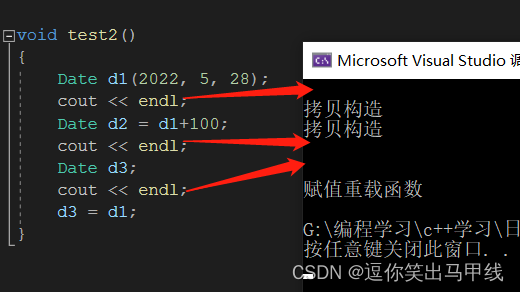### 3.3.3为什么赋值重载和拷贝构造函数里面的参数都建议用const修饰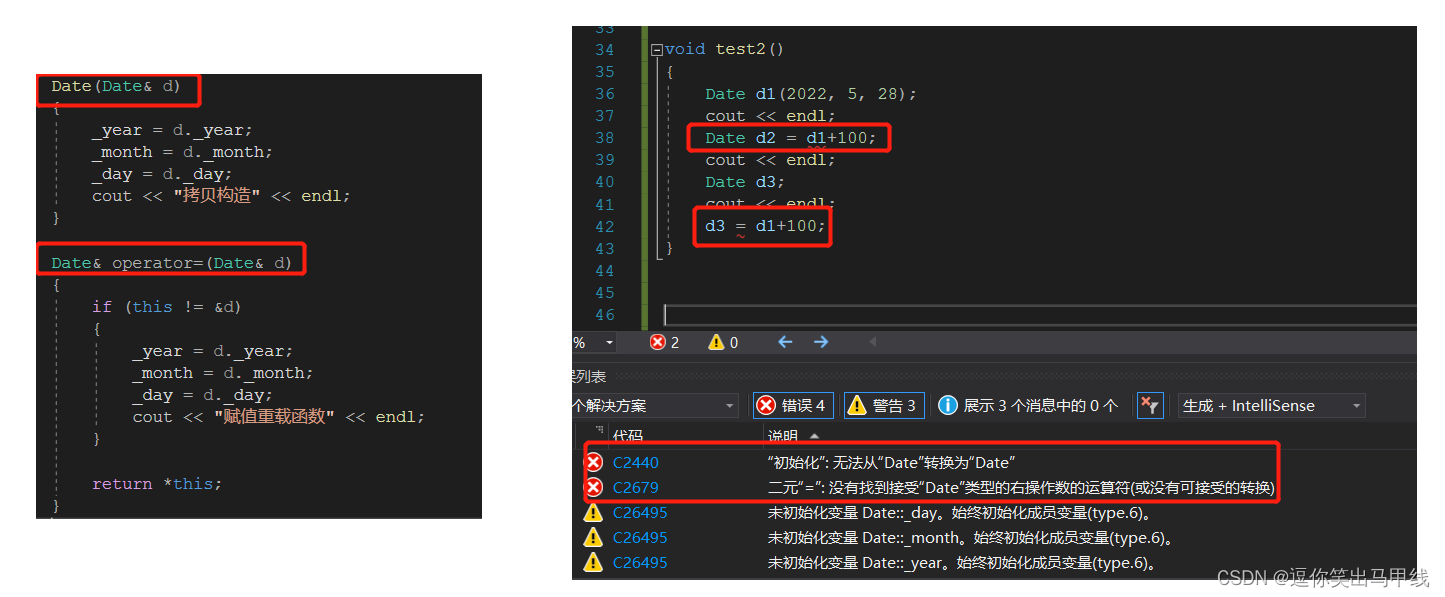程序报错了，为什么呢？我们来分析一下原因：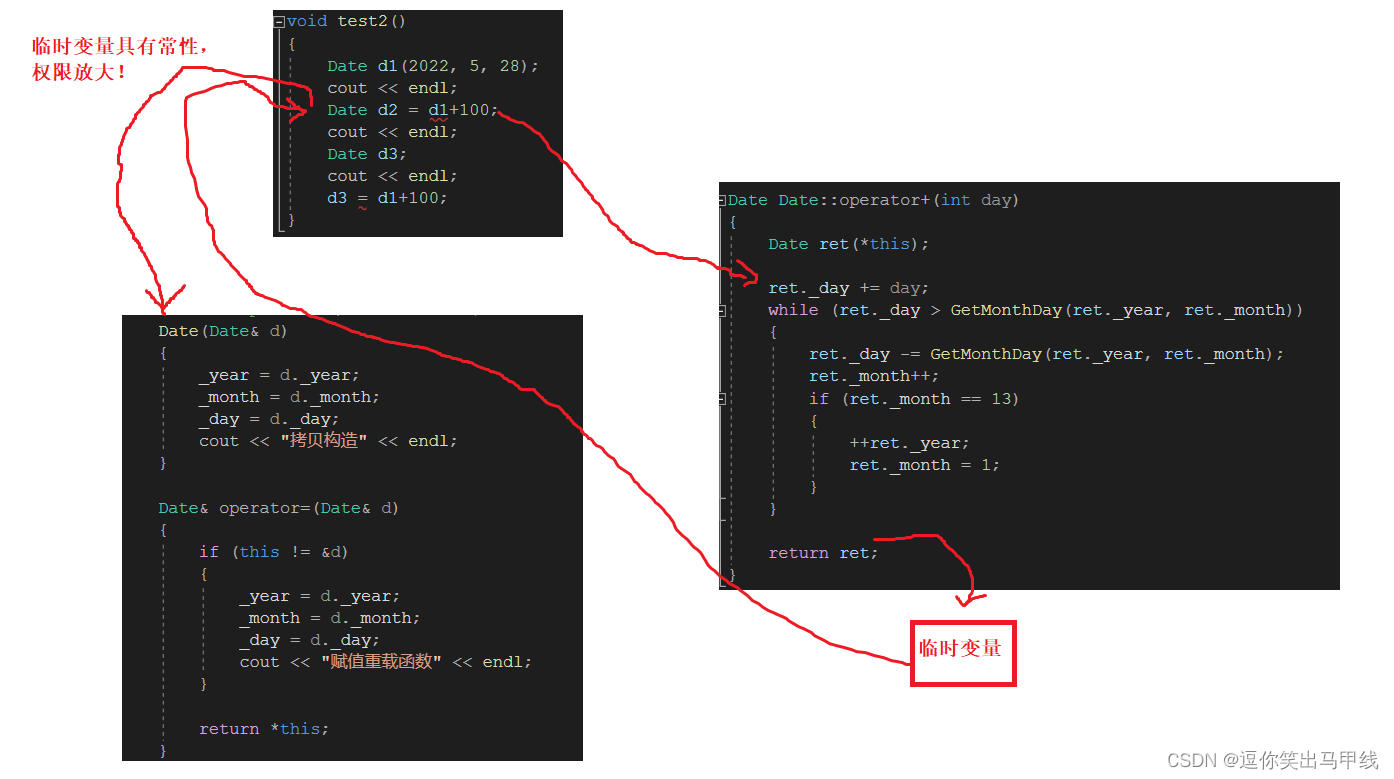因为在实现d1+100时，会调用operator+函数，返回ret时，由于他是一个类对象，返回时会生成一个临时对象，而临时对象具有常性，当d1+100作为右值赋值给d2时，调用拷贝构造函数，但是此时的拷贝构造函数的参数时Date&类型，不是const Date&，这相当于权限的放大，自然就会报错!

### 3.3.4"+="

+=和+的逻辑是一样的，但是+=之后原来的值会变，所以不需要返回临时变量，出了作用域this指向的内容也还在，这样我们用引用返回就可以了：

``Date& Date::operator+=(int day){if (day  GetMonthDay(_year, _month)){_day -= GetMonthDay(_year, _month);_month++;if (_month == 13){++_year;_month = 1;}}return *this;}``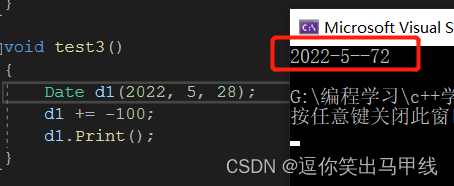### 3.3.5+和+=互相复用的优劣

``/*   +复用+=   */Date Date::operator+(int day) {Date ret(*this);ret += day;return ret;}Date& Date::operator+=(int day){if (day  GetMonthDay(_year, _month)){_day -= GetMonthDay(_year, _month);_month++;if (_month == 13){++_year;_month = 1;}}return *this;}/*   +=复用+   */Date Date::operator+(int day)  {Date ret(*this);ret._day += day;while (ret._day > GetMonthDay(ret._year, ret._month)){ret._day -= GetMonthDay(ret._year, ret._month);ret._month++;if (ret._month == 13){++ret._year;ret._month = 1;}}return ret;}Date& Date::operator+=(int day){*this = *this + day;return *this;}``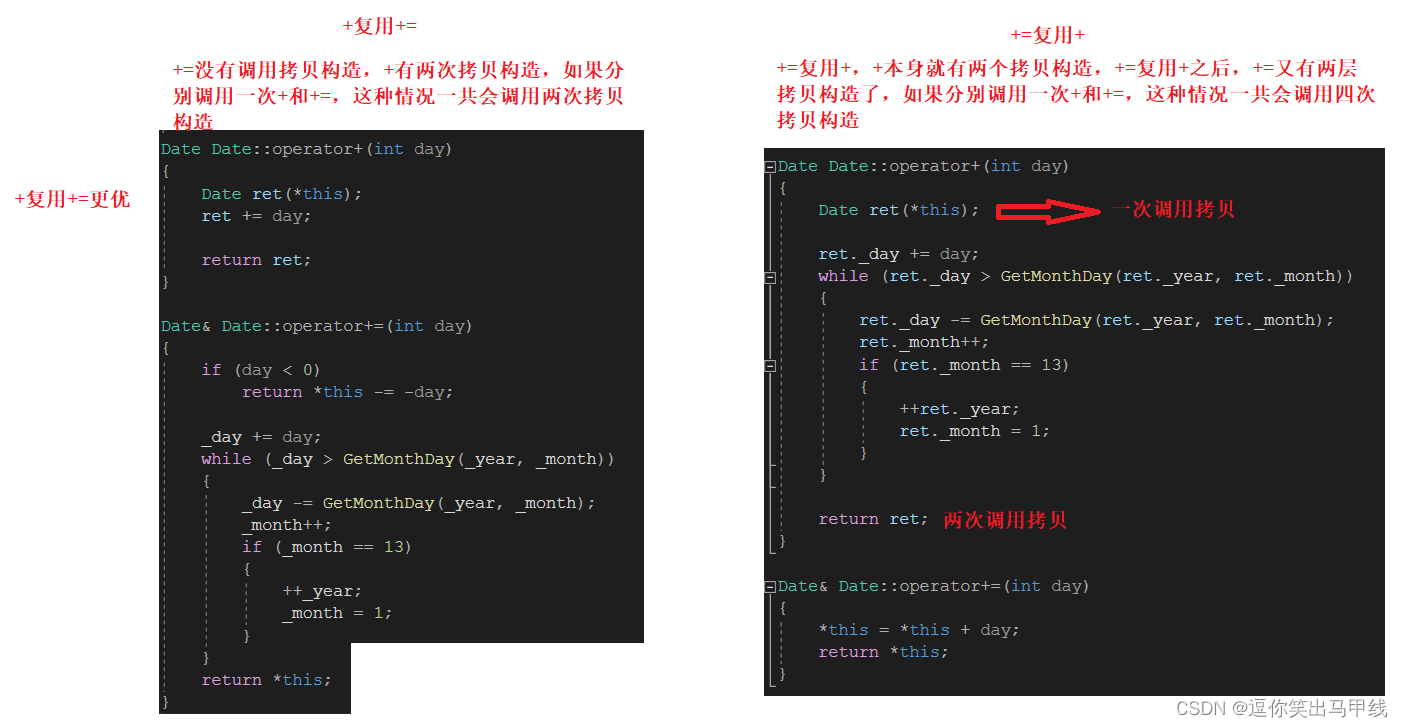## 3.4"-"和"-="

写完了+和+=，想必-和-=也很好些吧！代码如下：

``/*  -复用-=  */Date Date::operator-(int day) const{Date ret(*this);ret -= day;return ret;}Date& Date::operator-=(int day){if (day < 0)day = -day;_day -= day;while (_day <= 0){--_month;if (_month == 0){_month = 12;--_year;}_day += GetMonthDay(_year, _month);}return *this;}``

## 3.5前置++，--和后置++，--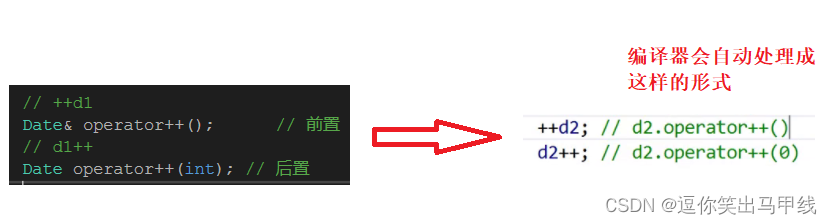那么我们如何实现前置++和后置++呢？首先我们要搞清楚，前置++是返回++后的值，后置++是返回++前的值。其代码如下：

``// ++d1Date& operator++()      // 前置{*this += 1;return *this;}// d1++Date operator++(int) // 后置{Date tmp(*this);*this += 1;return tmp;}``

``Date& operator--()     // 前置{*this -= 1;return *this;}Date operator--(int) // 后置{Date tmp(*this);*this -= 1;return tmp;}``

## 3.6"-"的另外一种重载形式，日期对象-日期对象

``int Date:: operator-(const Date& d) const{int sum = 0;int flag = 1;Date min = d;Date max = *this;if (min > max){flag = -1;min = *this;max = d;}while (min != max){max--;sum++;}return sum * flag;}``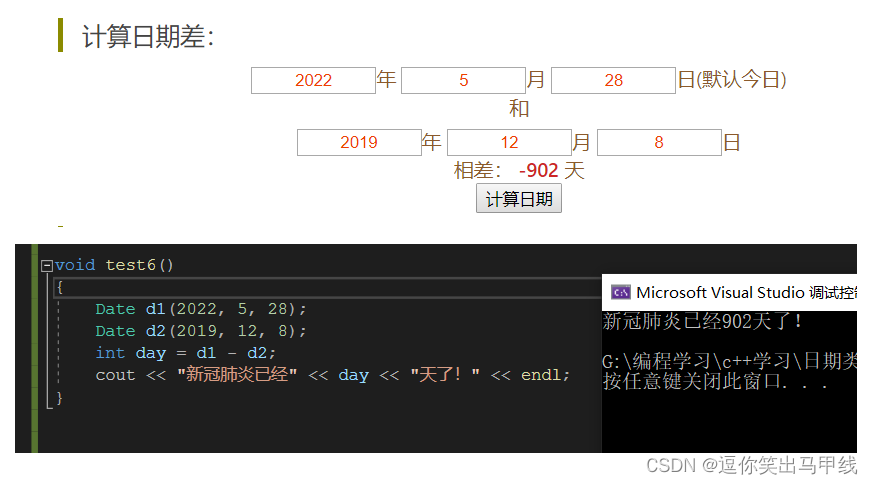结果是对的上的，我们写的代码没有错误。

## 3.7const修饰成员